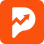Pocket Forex

# Moving Average (MA)

## What Is a Moving Average (MA)?

In statistics, a moving average is a calculation used to analyze data points by creating a series of averages of different subsets of the full data set. In finance, a moving average (MA) is a stock indicator that is commonly used in technical analysis. The reason for calculating the moving average of a stock is to help smooth out the price data by creating a constantly updated average price.

By calculating the moving average, the impacts of random, short-term fluctuations on the price of a stock over a specified time-frame are mitigated.

### KEY TAKEAWAYS

• A moving average (MA) is a stock indicator that is commonly used in technical analysis.
• The reason for calculating the moving average of a stock is to help smooth out the price data over a specified period of time by creating a constantly updated average price.
• A simple moving average (SMA) is a calculation that takes the arithmetic mean of a given set of prices over the specific number of days in the past; for example, over the previous 15, 30, 100, or 200 days.
• Exponential moving averages (EMA) is a weighted average that gives greater importance to the price of a stock on more recent days, making it an indicator that is more responsive to new information.

## Understanding Moving Average (MA)

Moving average is a simple, technical analysis tool. Moving averages are usually calculated to identify the trend direction of a stock or to determine its support and resistance levels. It is a trend-followingor laggingindicator because it is based on past prices.

The longer the time period for the moving average, the greater the lag. So, a 200-day moving average will have a much greater degree of lag than a 20-day MA because it contains prices for the past 200 days. The 50-day and 200-day moving average figures for stocks are widely followed by investors and traders and are considered to be important trading signals.

Moving averages are a totally customizable indicator, which means that an investor can freely choose whatever time frame they want when calculating an average. The most common time periods used in moving averages are 15, 20, 30, 50, 100, and 200 days. The shorter the time span used to create the average, the more sensitive it will be to price changes. The longer the time span, the less sensitive the average will be.

Investors may choose different time periods of varying lengths to calculate moving averages based on their trading objectives. Shorter moving averages are typically used for short-term trading, while longer-term moving averages are more suited for long-term investors.

There is no correct time frame to use when setting up your moving averages. The best way to figure out which one works best for you is to experiment with a number of different time periods until you find one that fits your strategy.

Predicting trends in the stock market is no simple process. While it is impossible to predict the future movement of a specific stock, using technical analysis and research can help you make better predictions.

A rising moving average indicates that the security is in an uptrend, while a declining moving average indicates that it is in a downtrend. Similarly, upward momentum is confirmed with a bullish crossover, which occurs when a short-term moving average crosses above a longer-term moving average. Conversely, downward momentum is confirmed with a bearish crossover, which occurs when a short-term moving average crosses below a longer-term moving average.

While calculating moving averages are useful in their own right, the calculation can also form the basis for other technical analysis indicators, such as the moving average convergence divergence (MACD).

The moving average convergence divergence (MACD) is used by traders to monitor the relationship between two moving averages. It is generally calculated by subtracting a 26-day exponential moving average from a 12-day exponential moving average.

When the MACD is positive, the short-term average is located above the long-term average. This an indication of upward momentum. When the short-term average is below the long-term average, this is a sign that the momentum is downward. Many traders will also watch for a move above or below the zero line. A move above zero is a signal to buy, while a cross below zero is a signal to sell.